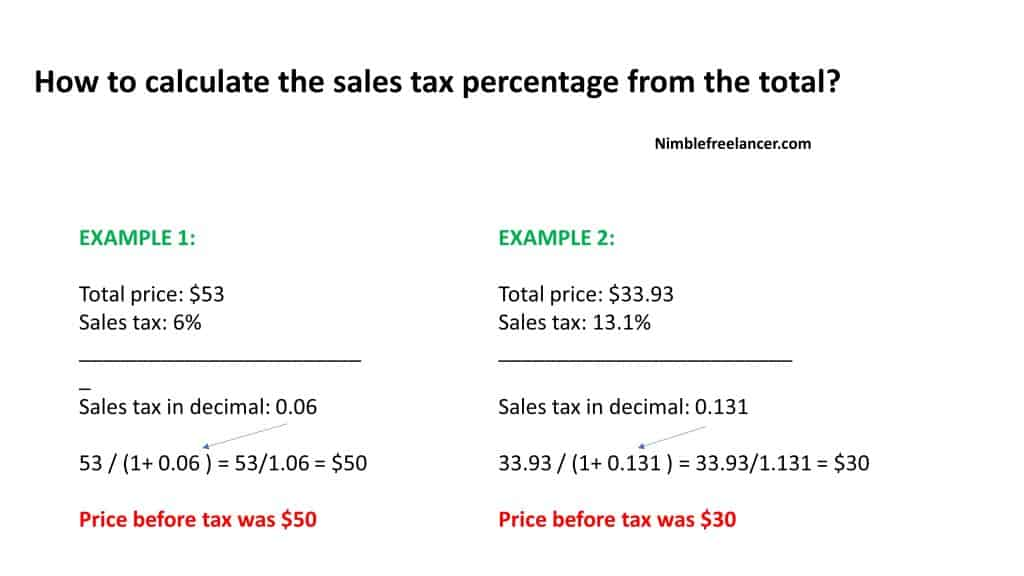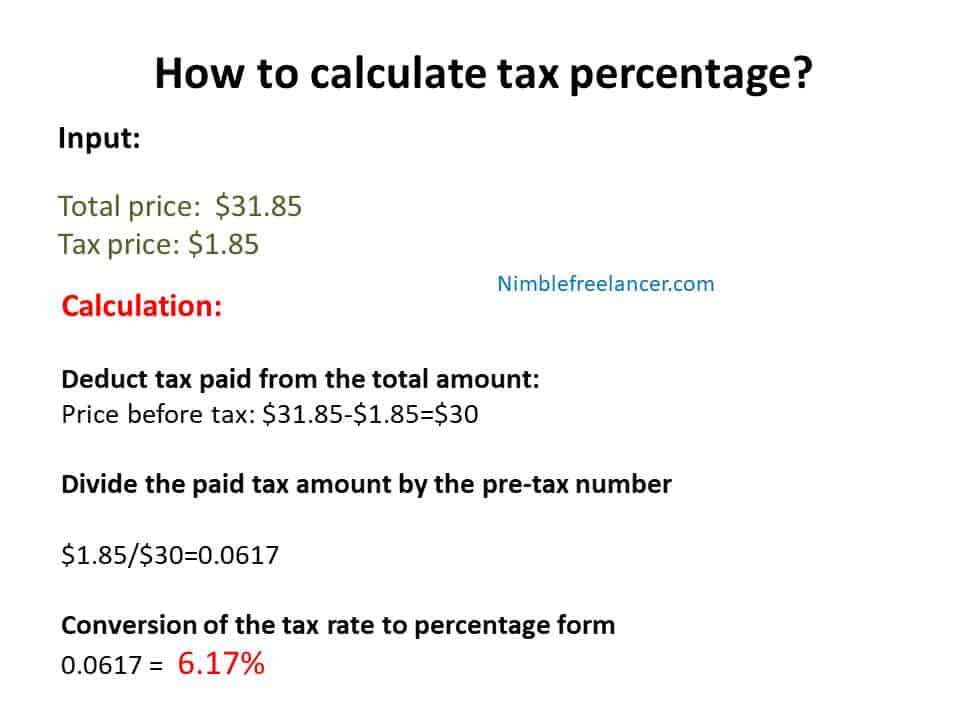# How to Calculate Sales Tax Backwards From Total? – Reverse Tax Calculator

A small but significant monetary compensation paid to the government as a response for the items and goods purchased is called a sales tax. The sellers usually collect this money to manage funds as part of the customers’ tax when purchased. The governments impose this rule in most countries, especially on services, facilities, and goods. However, these are conventional or retail sales taxes and only apply to the end-user who obtains the good.

## Reverse Tax calculator

[CP_CALCULATED_FIELDS id=”6″]

## How to calculate the sales tax percentage from the total?

To calculate the sales tax backward from the total, divide the total amount you received for the items subject to sales tax by “1 + the sales tax rate”. For example, if the sales tax rate is 5%, divide the total price by 1.05.You can calculate the tax paid by knowing the total post-tax price for the purchased item and the measured tax rate. In addition, this method can calculate the tax paid by determining it backward to analyze monetary compensation paid as sales tax.

The logic behind understanding sales tax relevance is the extensive documentation and investments in different manufacturing stages. Therefore various entities and companies ask for a slender deduction in monetary compensation, often equitable to sales tax.

It has become easier to understand and determine the cost margin for consumers and buyers. Keeping an insight and know-how regarding the sales track may facilitate retailers and buyers to check on the standard sales tax or value-added tax. It is imperative because sales tax is one of the most vital and fundamental financial institutions linked to the net business and retail exchange. In other words, sales tax is gradually but noticeably making a considerable impact on various purchase items, compelling the buyers to understand and analyze it at the time of purchase. With a mathematical formula, you can figure out the exact monetary percentage paid to the government. The same tax amount can be calculated with a straightforward multiplication of the net price, which is the original price, before applying sales tax with the sales tax percentage. The resulting amount is the total price, amalgamation of the net worth, and the sales tax.

This is an uncomplicated and straightforward way to determine the total cost, including the sales tax. By following a basic equation, you can keep track of the increasing tax rates, which are gradually becoming more significant. However, suppose you want to determine the amount of a purchased item preceding the tax application or calculate the sales tax unconventionally. The desired amount can be obtained with the same mathematical equation as the concept remains unchanged. You will need to know the total amount paid or the amount of tax applied for that purpose. By understanding these two determinants, you can effortlessly estimate the tax rate. If given the tax rate, you can quickly evaluate the amount of tax paid.

You can conclude the percentage tax rate if you know the total amount consumed and the total percentage of the paid tax amount.

## How to calculate tax percentage?

To calculate tax percentage, you need to subtract the tax paid from the total, divide the tax paid by the pre-tax price, and convert the tax rate to a percentage.• Step 1: Deduct tax paid from the total amount

You can withdraw the amount of tax you have paid from the total amount, which will be the good’s post-tax price. This will take you to the item’s price before the tax allocation; therefore, you will get the item’s pre-tax price. For example, if you have paid \$31.85 for two things and \$1.85 was the amount of tax applied as mentioned in the receipt, then the items’ cost before the tax was \$30. This amount has been derived after dividing the total cost for two things and the tax amount.

• Step 2: Divide the paid tax amount by the pre-tax number

You can also determine the sales tax by dividing the total amount of tax you have paid as a buyer by the item’s price before the tax. The resulting amount will be the percentage tax rate highlighted in a decimal form. For example dollar, 1.85÷30 dollars is equal to 0.0617.

• Step 3: Conversion of the tax rate to percentage form

There is another uncomplicated way to determine the sales tax. Multiply the mentioned tax rate by a hundred as it is a mathematical logic to convert numbers into percentage form. So if the tax rate result is 0.616 in decimal, you can convert it quickly into the percentage form. 0.0616× hundred is equal to 6.16%. Therefore 6.17% is the total tax rate you have paid on purchasing the two items.

## Adding 100 percent to the tax rate

In this case, you can calculate the total amount of tax paid by adding 100% to the actual sales tax rate. This hundred percent is synonymous with the pre-tax amount of the product purchased. When added to the tax rate, this whole amount will get you the total percentage that symbolizes or highlights the item’s pre-tax amount and the tax. For example, if an item has a sales tax of around 5%, you will get 105%.

## Conversion to decimal form

You can divide the tax-paid rate by a hundred to convert an amount to the decimal form. For example, if the total amount was 105%, you can divide by a hundred, giving you 1.05.

## Division of post-tax price with decimal

The purchased item’s post-tax price can be divided by the tax paid in a decimal form calculated by converting a percentage into a decimal form. That resulting amount will be before any amount of tax is applied. This is the pre-tax cost of the item, so if you have purchased an item that costs \$95 after the allocated taxes, you can divide it by 1.05 and get \$90.48.

## Subtraction of pre-tax price from the total or post-tax price

If you know the total cost of an item post and before the taxes, you can take out the pre-tax price and subtract it from the post-tax price to determine the exact amount of tax you have paid during retail. Therefore \$95 -\$90.48 is \$4.52. \$4.52 is the same amount of tax you have spent on a purchase.

Reverse sales tax is something you can easily calculate. However, if someone is interested in understanding the actual income and sales tax amount, you can also figure it backward and extract it from the total amount. Online reverse sales tax calculators make the process more accessible with the reverse sales tax formula. The formula is simple, dividing the sales receipt by one plus the sales tax percentage. Then, you can multiply the resulting amount by the tax rate and get a total sales tax dollar amount.

Latest posts by Daniel Smith (see all)

Daniel Smith

Daniel Smith is an experienced economist and financial analyst from Utah. He has been in finance for nearly two decades, having worked as a senior analyst for Wells Fargo Bank for 19 years. After leaving Wells Fargo Bank in 2014, Daniel began a career as a finance consultant, advising companies and individuals on economic policy, labor relations, and financial management. At Promtfinance.com, Daniel writes about personal finance topics, value estimation, budgeting strategies, retirement planning, and portfolio diversification. Read more on Daniel Smith's biography page. Contact Daniel: daniel@promtfinance.com